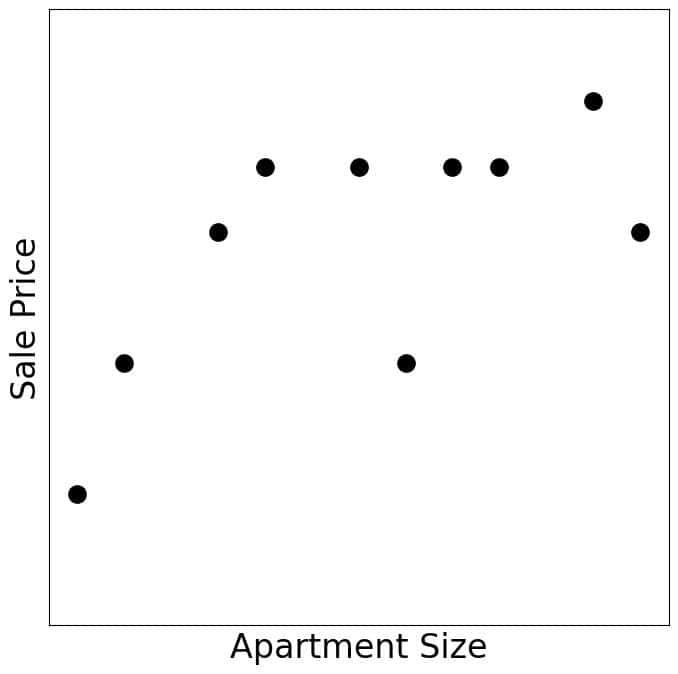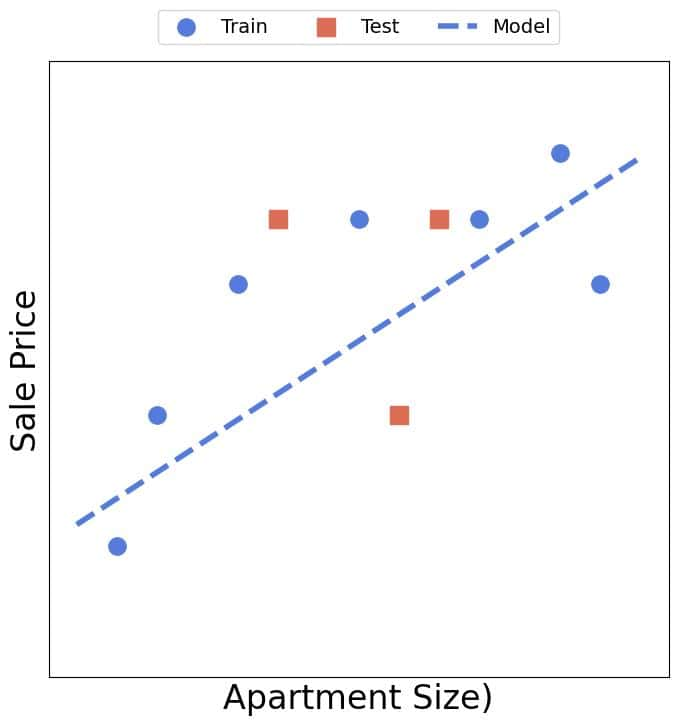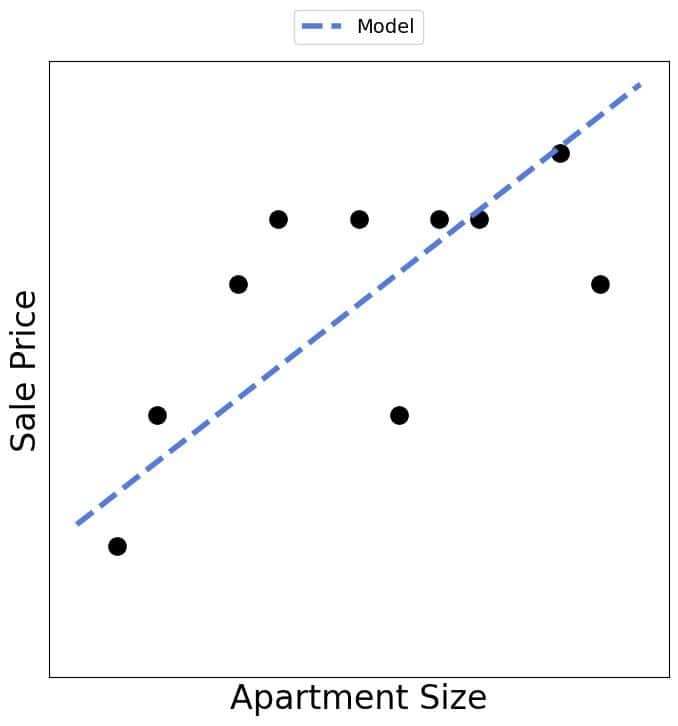## 1. Introduction

In this tutorial, we’ll talk about the differences between artificial intelligence, machine learning, statistics, and data mining.

There’s a significant overlap between those fields and no clear way to separate them. Over the years, researchers and engineers have articulated different and often conflicting opinions about this question, so there is no consensus.

## 2. Artificial Intelligence

Let’s start with artificial intelligence (AI). In general, the goal of AI is to build agents that can solve the problems we put before them on their own, as if they have innate intelligence like humans. What makes this field so rich is the abundance of ways to define and construct the agents.

For example, handwriting recognition software is an AI agent since it can read handwritten texts and convert them to a digital format without human help. It learns that ability from machine-readable images of handwritten digits and letters.

Another example is a chess-playing program. It plays chess by searching for the best possible sequence of moves considering what its opponent may play. The intelligence of such an AI agent doesn’t come from the data. Instead, it stems from how the agent searches for the best move.

There are other examples, such as sudoku solvers and airport flight schedules. The notion of an intelligent agent is at the center of them all. More precisely, we say that AI builds rational agents. Here, rationality means consistently choosing the best available option given what we know about all the alternatives: determining the word that best fits the handwriting, the move that will lead to winning a chess game the fastest, the flight schedule with the minimal waiting time, etc.

## 3. Machine Learning

Machine learning (ML) is a subfield of AI. Stepping back from the general AI terminology for a moment, we’d say that ML applies learning algorithms to datasets to get automated rules for predicting new data.

For instance, we may be interested in predicting the sale price of an apartment based. We have the data on various flats’ features (such as the size in square feet) and their final sale prices. Inducing a quality prediction rule from the dataset is a job for ML. Those rules may take different forms, such as trees, math equations in linear regression, or neural networks. So instead of working out the rules by hand, we use ML to extract them automatically.

But, given what we know about AI, we see that finding the ML rules falls under constructing AI agents. For example, we may restrict the price prediction rules to the equations of the form:

(1)Our goal is to discover the most accurate one. That’s the same as building an AI agent that, of all the prices it can predict using equation (1), outputs the one that is the most likely to be true. So, ML is a part of AI which builds the agents from data using the algorithms specialized for that.

## 4. Statistics

Unlike its relationship with AI, ML’s relationship with statistics is highly controversial. Many researchers, statisticians especially, would argue that ML is just a rebranded statistic. That argument is not without its merits. But, there are many researchers with a contesting view. To see why let’s first (try to) define statistics.

### 4.1. What Is Statistics?

Most people would describe statistics as the branch of mathematics for making inferences about a population using only a sample.

For example, we may want to discover the average height of teenagers in the USA. It would be impractical to measure all American teenagers. Instead, we could randomly select a few schools across the country and measure ten teenagers per school. That way, we’d get a sample of heights, the average of which informs us about the whole teenage population’s mean.

Similarly, we may be interested to see how temperature affects an industrial process or how the number of rooms in a flat influences its sale price.

Statistics develops the methods for answering such questions. In doing so, it is highly formal. All its tools, such as hypothesis testing and descriptive statistics, come with mathematical proofs of performance. For instance, we know that theconfidence intervals we construct around the sample means are guaranteed to capture the actual population meanof the time. Such proofs, however, rely on assumptions that may not hold in reality. For example, common assumptions are statistical independence of the sample elements and normality of data.

### 4.2. Why Machine Learning Is Statistics?

The researchers in favor of this view argue that inducing a prediction rule (of any form) from data is nothing else than making an inference about the process producing those data. For instance, the equation ML gives us to predict flat prices is also an inference about the general rule that the whole “population” of apartments follows on sale. Likewise, inferring a general rule about a data generating process in statistics allows predicting new data.

In support of this argument, people also say that some core ML models like linear regression were initially developed and researched in statistics. They go a step further and assert that all ML models are statistical tools. The only difference is that the former are less interpretable and computationally more demanding. Even more so, some researchers voice the concern that ML is statistics done wrong. The reason is that automated modeling lacks proper rigor possible only through human involvement.

### 4.3. Why Machine Learning Is Not Statistics?

But, many ML researchers would strongly oppose those claims. They would argue that the focus on prediction makes ML different from statistics. Most of the time, especially in industry, performance metrics are all that counts. So, a deep neural network with hundreds of inner layers is a perfectly acceptable ML product if its predictions are accurate even though, by itself, it isn’t interpretable and doesn’t allow for any inference. On the other hand, a statistician would be very uncomfortable using a black-box model like that.

Further, since ML focuses on predictive performance, it validates its models on held-out test data to check their generalization capabilities. Statistics, however, don’t split samples into training and test sets.

Additionally, it seems that ML pays attention to the engineering and computational aspects of training its models and handling large datasets much more than statistics. The reason is that statisticians developed their tools to work on small samples precisely to avoid dealing with large amounts of data. In contrast, ML tools originated from the computer science and AI fields, so the scientists considered the algorithmic and their implementation aspects from the start. As a result, the ML methodology replaced the statistical one as it solved the tasks that traditional statistics couldn’t tackle.

An interesting argument is that the research cultures of ML scientists and statisticians differ. Extensive theoretical results accompany all statistical methods. For instance, it’s impossible to publish a paper about a new statistical test without theorems and proofs. The empirical evaluation alone wouldn’t cut it. In contrast, ML researchers and practitioners would show interest in a method with good results on real-world data even though there may be gaps in its theory.

### 4.4. Example

Finally, although we can use ML and statistics for both prediction and inference, their methodologies differ. ML isn’t just reworded statistics done wrong. It employs a different modeling approach.

For example, let’s say that a dataset contains the sizes (in square feet) and sale prices of ten apartments:To fit a linear model, an ML scientist would divide the set into training (blue) and test (red) data and minimize the loss over the former:Then, the s(he) would evaluate the model on the three test data. If the errors are negligible and not that different from those on the training data, the scientist would consider it a good model for predicting the price based on the apartment size.

In contrast, a statistician would fitto the whole dataset (probably obtaining different coefficients):But, s(he) wouldn’t use it for prediction. Instead, s(he) would test the hypothesis that the size affects the sale price by checking the significance of the coefficient.

This example may present an overly simplistic view of the two disciplines in practice but illustrates the difference between the approaches.

## 5. Data Mining

Data mining grew out of database management in commercial applications. Its goal is to discover valuable patterns in big data and provide business stakeholders with actionable information. Let’s illustrate that with an example.

### 5.1. Example

Let’s say that an online news agency wants to find from where its audience comes. It needs that information to focus on covering the stories on the regions where the majority of its active subscribers live. The site cares only about the people who have already subscribed to its feed and often read the news, not those that might do so in the future or are passive subscribers.

That’s the opposite of what we do in statistics with inferences and machine learning with predictions. A statistician would define a hypothesis about the proportions of subscribers living in the predefined set of regions. Then, (s)he would select the appropriate statistical test and check if the data meets its assumptions. If not, the statistician would not proceed further, informing us the data don’t allow for valid inference. Otherwise, (s)he would run the test and tell us if it rejected or failed to reject the hypothesis.

But, statistical hypotheses are about populations, not samples. Even if our statistician has anything to tell us, that would be about the people like our current active subscribers, i.e., our potential audience of interest. However, it isn’t the information we need as we want to tailor the editorial policy to current readers.

Similarly, an ML model could predict, for each region, the probability that a random person from it would be interested in subscribing to the site. Again, although nice to have, the model isn’t what we want. As our example shows, mining is often about getting insight into the dataset at hand, ignoring everything out of it. In other words, data miners find the patterns in the given data, not the population from which we sampled the dataset. In fact, in most cases, the data contain the whole population of interest.

### 5.2. But Hey, Isn’t Data Mining Just Applied Statistics and Machine Learning?

As with ML and statistics, some would argue that data mining is just applied statistics or applied ML (in particular, unsupervised). After all, that’s where the tools it uses originated. So, if it is just about applying other fields’ methods, can we consider mining a discipline of its own? Many statisticians and ML researchers would argue we shouldn’t. But, many data miners oppose this view, highlighting the differences we discussed above.

Additionally, data mining prioritizes results over methodology. Heuristic approaches are acceptable as long as they provide value to the business, even though they may not be well-founded mathematically. Further, miners sometimes devise an analysis method specifically for the data at hand and don’t bother with its applicability to other datasets.

Finally, the emphasis is on exploring the data. So, mining is an exploratory activity with specific goals set ahead. Although ML and statistics do exploratory analysis, they focus on checking assumptions, not extracting business-critical information.

## 6. Conclusion

In this article, we talked about artificial intelligence, machine learning, statistics, and data mining. There is no consensus on the boundaries between the four fields. In particular, some consider the latter three the variations of the same discipline. However, although the lines are blurred, we can spot their differences.